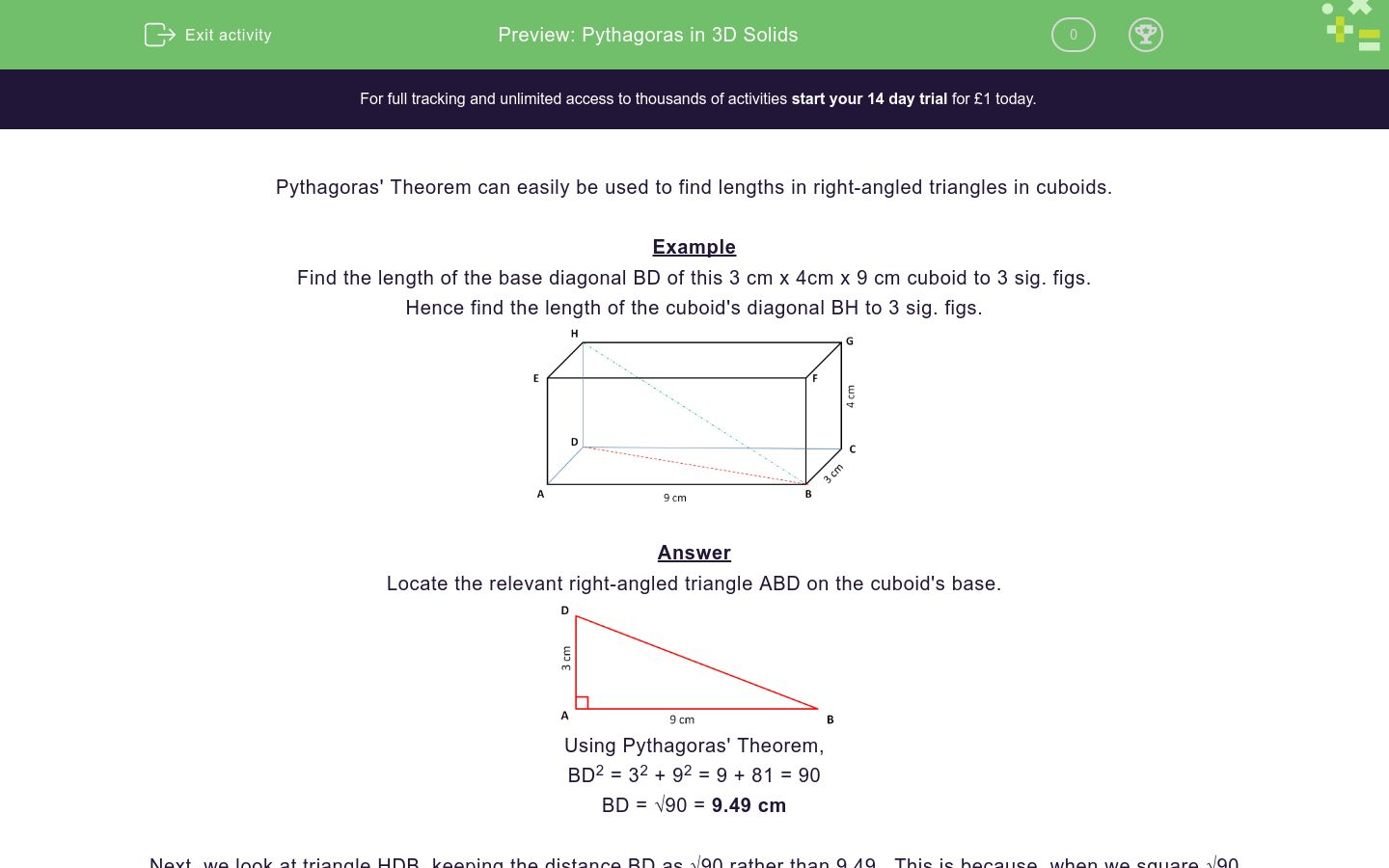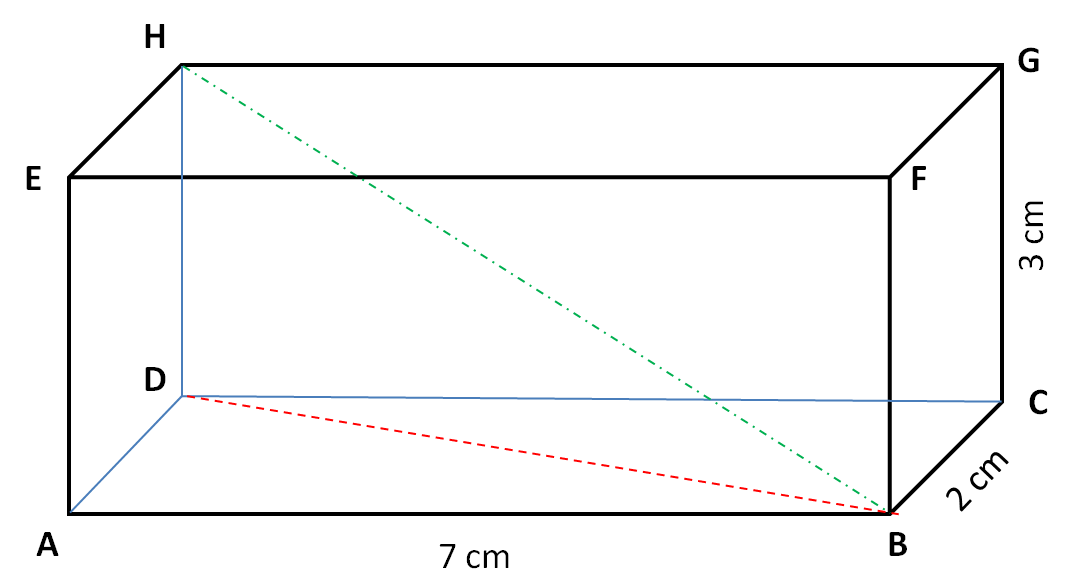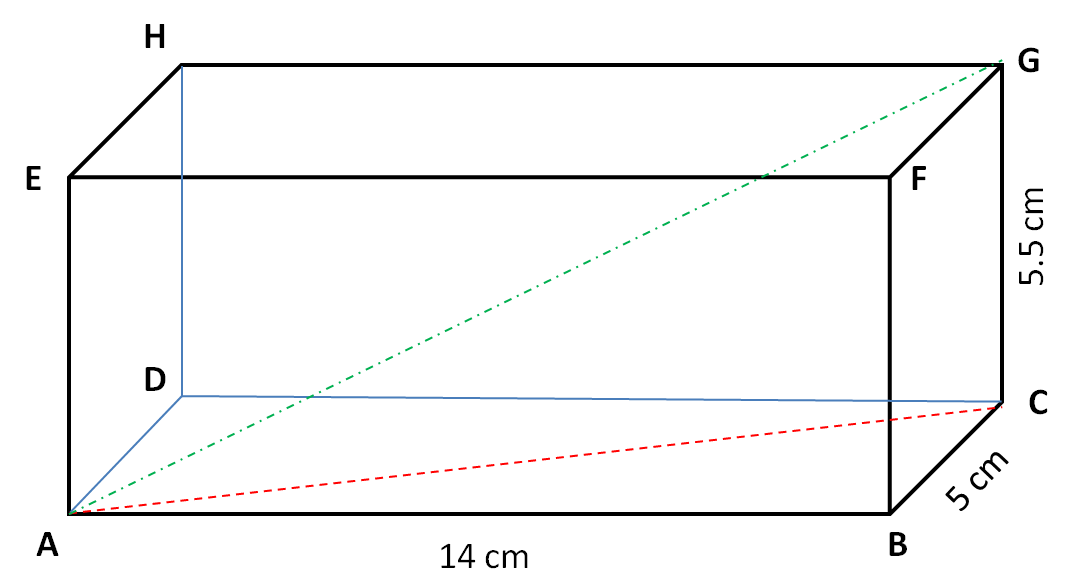# Pythagoras in 3D Solids

In this worksheet, students use Pythagoras' Theorem to calculate lengths in cuboids.Key stage:  KS 4

Curriculum topic:  Geometry and Measures

Difficulty level:### QUESTION 1 of 10

Pythagoras' Theorem can easily be used to find lengths in right-angled triangles in cuboids.

Example

Find the length of the base diagonal BD of this 3 cm x 4cm x 9 cm cuboid to 3 sig. figs.

Hence find the length of the cuboid's diagonal BH to 3 sig. figs.Locate the relevant right-angled triangle ABD on the cuboid's base.Using Pythagoras' Theorem,

BD2 = 32 + 92 = 9 + 81 = 90

BD = √90 = 9.49 cm

Next, we look at triangle HDB, keeping the distance BD as √90 rather than 9.49.  This is because, when we square √90 we get exactly 90.Using Pythagoras' Theorem,

BH2 = 42 + (√90)2 = 16 + 90 = 106

BH = √106 = 10.3 cm

Alternative Method

An alternative method is to say that:

BH2 = HD2 + AD2 + AB2 = 42 + 32 + 92 = 16 + 9 + 81 = 106

BH = √106 = 10.3 cm

Find the length of the base diagonal BD of this 2 cm x 3 cm x 7 cm cuboid in cm to 3 sig. figs.

(Just write the number)Find the length of the cuboid's diagonal BH of this 2 cm x 3 cm x 7 cm cuboid in cm to 3 sig. figs.

(Just write the number)Find the length of the base diagonal BD of this 3 cm x 5 cm x 11 cm cuboid in cm to 3 sig. figs.

(Just write the number)Find the length of the cuboid's diagonal BH of this 3 cm x 5 cm x 11 cm cuboid in cm to 3 sig. figs.

(Just write the number)Find the length of the base diagonal BD of this 4 cm x 5 cm x 12 cm cuboid in cm to 3 sig. figs.

(Just write the number)Find the length of the cuboid's diagonal BH of this 4 cm x 5 cm x 12 cm cuboid in cm to 3 sig. figs.

(Just write the number)Find the length of the base diagonal AC of this 4 cm x 5 cm x 10 cm cuboid in cm to 3 sig. figs.

(Just write the number)Find the length of the cuboid's diagonal AG of this 4 cm x 5 cm x 10 cm cuboid in cm to 3 sig. figs.

(Just write the number)Find the length of the base diagonal AC of this 5 cm x 5.5 cm x 14 cm cuboid in cm to 3 sig. figs.

(Just write the number)Find the length of the cuboid's diagonal AG of this 5 cm x 5.5 cm x 14 cm cuboid in cm to 3 sig. figs.

(Just write the number)• Question 1

Find the length of the base diagonal BD of this 2 cm x 3 cm x 7 cm cuboid in cm to 3 sig. figs.

(Just write the number)7.28
EDDIE SAYS
Use Pythagoras' Theorem to get:
BD² = 2² + 7² = 4 + 49 = 53
• Question 2

Find the length of the cuboid's diagonal BH of this 2 cm x 3 cm x 7 cm cuboid in cm to 3 sig. figs.

(Just write the number)7.87
EDDIE SAYS
Use Pythagoras' Theorem to get:
BH² = 2² + 7² + 3² = 4 + 49 + 9 = 62
• Question 3

Find the length of the base diagonal BD of this 3 cm x 5 cm x 11 cm cuboid in cm to 3 sig. figs.

(Just write the number)11.4
EDDIE SAYS
Use Pythagoras' Theorem to get:
BD² = 3² + 11² = 9 + 121 = 130
• Question 4

Find the length of the cuboid's diagonal BH of this 3 cm x 5 cm x 11 cm cuboid in cm to 3 sig. figs.

(Just write the number)12.4
EDDIE SAYS
Use Pythagoras' Theorem to get:
BH² = 3² + 5² + 11² = 9 + 25 + 121 = 155
• Question 5

Find the length of the base diagonal BD of this 4 cm x 5 cm x 12 cm cuboid in cm to 3 sig. figs.

(Just write the number)12.6
EDDIE SAYS
Use Pythagoras' Theorem to get:
BD² = 12² + 4² = 144 + 16 = 160
• Question 6

Find the length of the cuboid's diagonal BH of this 4 cm x 5 cm x 12 cm cuboid in cm to 3 sig. figs.

(Just write the number)13.6
EDDIE SAYS
Use Pythagoras' Theorem to get:
BH² = 4² + 5² + 12² = 16 + 25 + 144 = 185
• Question 7

Find the length of the base diagonal AC of this 4 cm x 5 cm x 10 cm cuboid in cm to 3 sig. figs.

(Just write the number)10.8
EDDIE SAYS
Use Pythagoras' Theorem to get:
AC² = 4² + 10² = 16 + 100 = 116
• Question 8

Find the length of the cuboid's diagonal AG of this 4 cm x 5 cm x 10 cm cuboid in cm to 3 sig. figs.

(Just write the number)11.9
EDDIE SAYS
Use Pythagoras' Theorem to get:
AG² = 4² + 5² + 10² = 16 + 25 + 100 = 141
• Question 9

Find the length of the base diagonal AC of this 5 cm x 5.5 cm x 14 cm cuboid in cm to 3 sig. figs.

(Just write the number)14.9
EDDIE SAYS
Use Pythagoras' Theorem to get:
AC² = 5² + 14² = 25 + 196 = 221
• Question 10

Find the length of the cuboid's diagonal AG of this 5 cm x 5.5 cm x 14 cm cuboid in cm to 3 sig. figs.

(Just write the number)